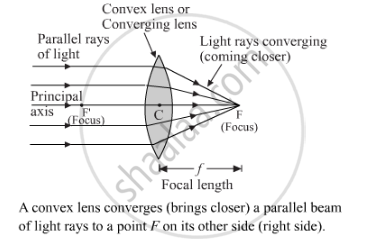Share

# Which Type of Lens is : a Converging Lens, and Which is - Science

Course

#### Question

Which type of lens is :

a converging lens, and which is

#### Solution

A lens that converges a parallel beam of light passing through it to a point is known as a converging lens. The convex lens is a converging lens. The ray diagram below shows the parallel light rays converging at the focus of a convex lens.Is there an error in this question or solution?